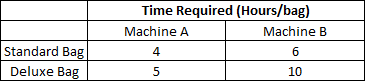Question 59

# A leather factory produces two kinds of bags, standard and deluxe. The profit margin is Rs. 20 on a standard bag and Rs. 30 on a deluxe bag. Every bag must be processed on machine A and on Machine B. The processing times per bag on the two machines are as follows:The total time available on machine A is 700 hours and on machine B is 1250 hours. Among the following production plans, which one meets the machine availability constraints and maximizes the profit?

Solution

.Let x be no. of standard bags and y be no. of deluxe bags. According to given conditions we have 2 equations 4x+5y<=700 and 6x+10y<=1250. Here option A satisfies both the equations.

• All Quant CAT Formulas and shortcuts PDF
• 30+ CAT previous papers with solutions PDF